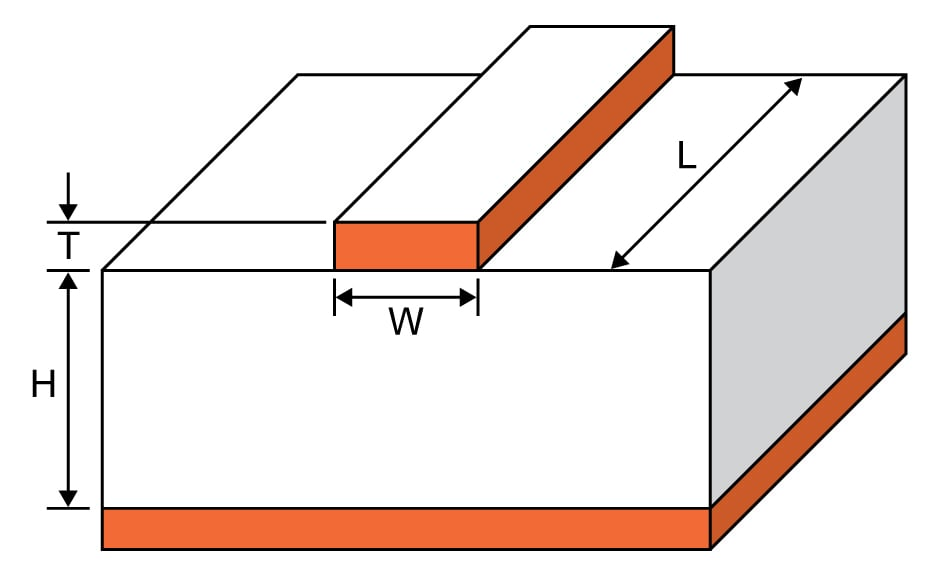# Microstrip Inductance Calculator

## This calculator computes the inductance of a microstrip etched on a PCB.

Inches
Inches
Inches

μH

### Overview

A microstrip is created by etching a trace on a printed circuit board and is mostly used in microwave applications. This calculator is designed to calculate the inductance of a microstrip given its dimensions (width, length and the distance of the strip from the ground plane).### Equation

$$L_{ms}= 0.00508L\left [ ln \left ( \frac{2L}{W+H} \right )+0.5+0.2235\frac{(W+H)}{L} \right ]$$

where:

$$L_{ms}$$ = inductance of the microstrip in microhenries (μH)

$$W$$ =  width of the strip in inches

$$L$$ = length of the strip in inches

$$H$$ = distance between the strip and the ground plane

### Applications

A microstrip is a flat conductor separated by insulating dielectric from a large conducting ground plane. The microstrip is designed so that its length is one-quarter or one-half of the wavelength of the signal propagating through it. Microstrips arose because, at very high frequencies, the required circuit inductance and capacitance values were too small for standard inductors and capacitors. As such, microstrips serve the same function as inductors and capacitors only at microwave frequencies. They are used as tuned circuits, impedance-matchers, filters, phase shifters, and reactive components.